##### TI-84 Plus CE Graphing Calculator For Dummies, 3rd EditionThe Equation Solver on your TI-84 Plus calculator is a great tool for solving one-variable equations. The Solver is also capable of solving an equation for one variable given the values of the other variables. Keep in mind that the Solver can only produce real-number solutions.

After you have had experience using the Solver, you can refer back to this list, if necessary, to refresh your memory on its use.

1. Enter a new equation in the Equation Solver.

2. Enter a guess for the solution.

3. Press [ALPHA][ENTER] to solve the equation.

## Step 1: Enter or edit the equation to be solved

For this exercise, you're going to use the Equation Solver to solve the equation, 2(3 X) = 4X 7. To enter an equation in the Solver, follow these steps:

1. Access the Solver from the Math menu by pressing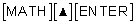When the Solver appears, it should look similar to the first screen.

The Solver in the TI-84 Plus works a little differently. Set the original equation equal to zero and enter the resulting equation into the Solver.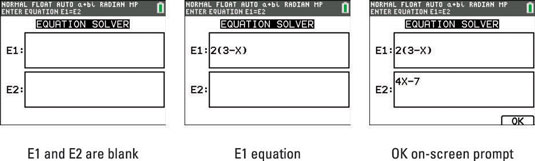2. Enter the left side of the equation to be solved in E1.

If equation E1 already contains an equation, press C before entering the left side of the equation to be solved. See the second screen.

3. Press the down-arrow key and enter the right side of the equation to be solved in E2.

If equation E2 already contains an equation, press [CLEAR] before entering the right side of the equation to be solved.

4. Press [GRAPH] to activate the on-screen OK prompt.

Notice that the on-screen OK prompt does not appear until you enter expressions in both E1 and E2. See the third screen.

You can also use a function that you’ve entered in the Y= editor in the definition of your equation. To insert such a function into the E1 or E2, press [ALPHA][TRACE] to access the Y-variables menu and then press the number of the Y-variable you want to enter.

## Step 2: Guess a solution

Guess at a solution. Any value in the interval defined by the bound variable will do. Guessing is necessary because your calculator solves problems through an iterative process. The bound variable at the bottom of the screen (see the first screen) is where you enter the bounds of the interval containing the solution you’re seeking. The default setting for this interval is [1099, 1099], as is indicated by bound = {1E99, 1E99}.

1E99 is (1*1099) in scientific notation. That’s a huge number!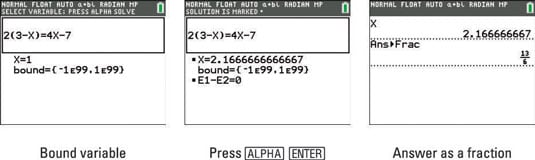If your guess is close to the solution, the calculator quickly solves the equation; if it’s not, it may take the calculator a while to solve the equation.

If your equation has more than one solution, the calculator will find the one closest to your guess.

## Step 3: Solve the equation

To solve an equation, follow these steps:

1. To place the cursor anywhere in the line that contains the variable you’re solving for, use the following keys: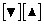Place your cursor in the variable for which you want to make a guess.

2. Press [ALPHA][ENTER] to solve the equation.

The second screen shows this procedure; the square indicator shown next to the X indicates that X is the variable just solved for.

You can access the calculated solution on the Home screen. Press [2nd][MODE] to quit the application. Next, type the variable you solved for, in this case, X. To see your answer in decimal form, press the following key followed by [ENTER]: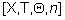Press [MATH][ENTER][ENTER] to convert your answer to a fraction. See the third screen.

The E1 – E2 value that appears at the bottom of the second screen evaluates the two sides of the equation (using the values assigned to the variables) and displays the difference — that is, the accuracy of this solution. An E1 – E2 value of zero indicates an exact solution.

If you get the ERR: NO SIGN CHNG error message when you attempt to solve an equation using the Equation Solver, then the equation has no real solutions in the interval defined by the bound variable.